# Transient Magnetic : solved equations (vector potential 3D)

## Introduction

The magnetic vector potential is a proposed model for 3D applications.

This model can be used by :

• The 3D solver of Flux 3D.

## Equation solved with magnetic vector potential model (3D)

The Gauss law for magnetism,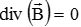,

allows the introduction of a magnetic vector potential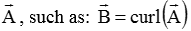The equation solved by the finite elements method in a Transient Magnetic application is written: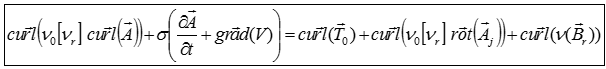Where:

r] is the tensor of the reluctivity of the medium

ν0 is the magnetic reluctivity of the vacuum ; ν0 = 1/μ0 = 1/(4 π 10-7) (in m/H)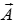is the magnetic vector potential (in Wb/m)

σ is the electrical conductivity of the medium (in S)

V is the magnetic scalar potential (in A/m²)is the source electric vector potential source (in A/m)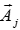is the source magnetic vector potential (in Wb/m)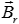is the induction of the magnets (in Wb/m)

## Use of formulations

This new formulation is particularly appreciated in the context of calculation of eddy currents (induction heating, induction motor...), on solid conductor type regions. In this context, it provides more accurate results and has a better convergence than the current formulation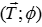.

These two formulations are dual and therefore each have their advantages, the formulationis more accurate when calculating induction at the expense of current density.

These new formulations don't need electrical or magnetic cut.

Warning, these formulations are only available when there is no mechanical set.

The previous formulation AWVT0W is still usable but not available when selecting new formulations.

## The formulations in Flux

Problems

Formulations (in Flux 3D)

Solid conductors with unmeshed coils coupled circuit or not and meshed coils coupled circuits or not. AWVT0WAJW
Other regions AWT0WAJW

## Comparison

A comparison has been made to ensure the reliability of these new formulations. The test is performed on an induction heating problem whose results are available in the figures below.Formulation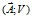Formulation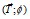Calculation time (s) 477.38 1143.80
Average number of iterations of the nonlinear solver. 21 26
Average relative error (%) 4.61